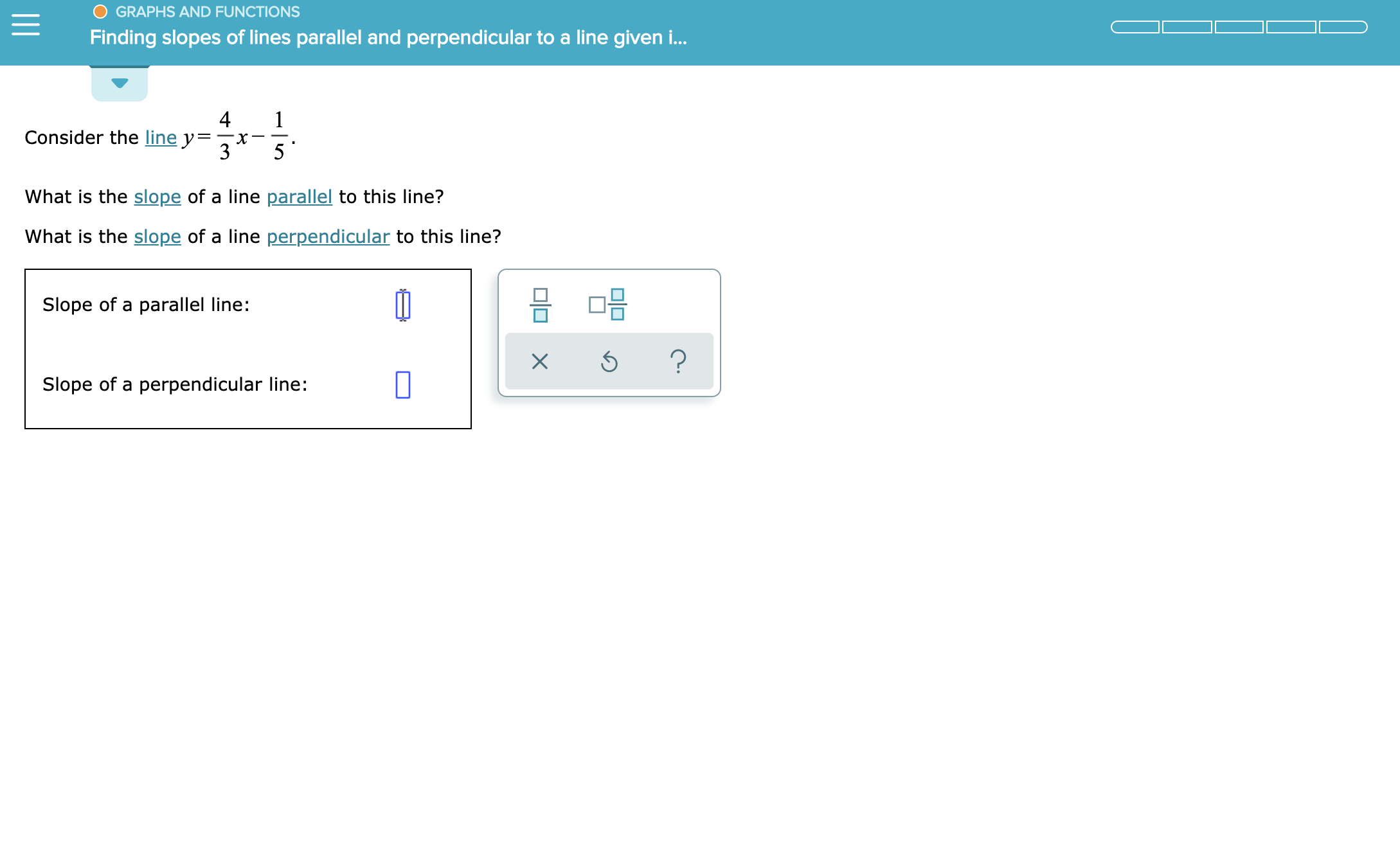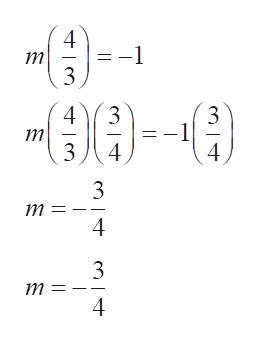# GRAPHS AND FUNCTIONSFinding slopes of lines parallel and perpendicular to a line given i...4Consider the line y=315What is the slope of a line parallel to this line?What is the slope of a line perpendicular to this line?믐 □믐Slope of a parallel line:?XSlope of a perpendicular line:

Question
3 views

See attachedhelp_outlineImage TranscriptioncloseGRAPHS AND FUNCTIONS Finding slopes of lines parallel and perpendicular to a line given i... 4 Consider the line y= 3 1 5 What is the slope of a line parallel to this line? What is the slope of a line perpendicular to this line? 믐 □믐 Slope of a parallel line: ? X Slope of a perpendicular line: fullscreen
check_circle

Step 1

Given line is:

Equation of a line in slope-intercept form is y = mx + c, where m is the slope and c is the intercept.

Comparing to the given equation, slope and y – intercept are given by:

Step 2

When two lines are parallel, the slopes will be equal.

Hence, the slope of a line parallel to the given line is:

Step 3

When two lines are perpendicular, the product of slopes is -1.

Let the slope of the perpendicular line to the give...help_outlineImage Transcriptionclose4 =-1 3 4 3 3 4 4 3 m = 4 3 m = 4 fullscreen

### Want to see the full answer?

See Solution

#### Want to see this answer and more?

Solutions are written by subject experts who are available 24/7. Questions are typically answered within 1 hour.*

See Solution
*Response times may vary by subject and question.
Tagged in

### Calculus